# 1.6 Components of a vector  (Page 4/4)

 Page 4 / 4

Write force OA in component form.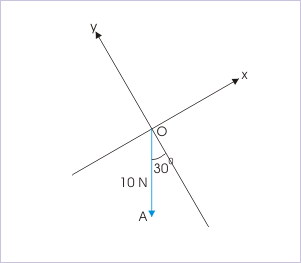Component of a vector.

${F}_{x}=10\mathrm{sin}{30}^{0}=10X\frac{1}{2}=5\phantom{\rule{1em}{0ex}}N$ ${F}_{y}=10\mathrm{cos}{30}^{0}=10X\frac{\sqrt{3}}{2}=5\sqrt{3}\phantom{\rule{1em}{0ex}}N$ $\mathbf{F}=-{F}_{x}\mathbf{i}-{F}_{y}\mathbf{j}=-5\mathbf{i}-5\sqrt{3}\mathbf{j}\phantom{\rule{1em}{0ex}}N$

Write force OA in component form.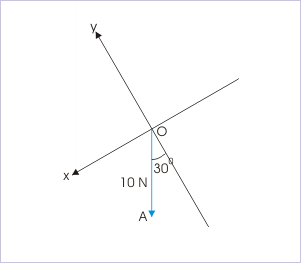Component of a vector.

${F}_{x}=10\mathrm{sin}{30}^{0}=10X\frac{1}{2}=5\phantom{\rule{1em}{0ex}}N$ ${F}_{y}=10\mathrm{cos}{30}^{0}=10X\frac{\sqrt{3}}{2}=5\sqrt{3}\phantom{\rule{1em}{0ex}}N$ $\mathbf{F}={F}_{x}\mathbf{i}-{F}_{y}\mathbf{j}=5\mathbf{i}-5\sqrt{3}\mathbf{j}\phantom{\rule{1em}{0ex}}N$

## Vector addition : algebraic method

Graphical method is meticulous and tedious as it involves drawing of vectors on a scale and measurement of angles. More importantly, it does not allow algebraic operations that otherwise would give a simple solution. We can, however, extend algebraic techniques to vectors, provided vectors are represented on a rectangular coordinate system . The representation of a vector on coordinate system uses the concept of unit vector and component.

Now, the stage is set to design a frame work, which allows vector addition with algebraic methods. The frame work for vector addition draws on two important concepts. The first concept is that a vector can be equivalently expressed in terms of three component vectors :

$\begin{array}{l}\mathbf{a}={a}_{x}\mathbf{i}+{a}_{y}\mathbf{j}+{a}_{z}\mathbf{k}\\ \mathbf{b}={b}_{x}\mathbf{i}+{b}_{y}\mathbf{j}+{b}_{z}\mathbf{k}\end{array}$

The component vector form has important significance. It ensures that component vectors to be added are restricted to three known directions only. This paradigm eliminates the possibility of unknown direction. The second concept is that vectors along a direction can be treated algebraically. If two vectors are along the same line, then resultant is given as :

When θ = 0°, cosθ = cos 0° = 1 and,

$\begin{array}{l}⇒R=\surd \left({P}^{2}+2PQ+{Q}^{2}\right)=\surd \left\{{\left(P+Q\right)}^{2}\right\}=P+Q\end{array}$

When θ = 180°, cosθ = cos 180° = -1 and,

$\begin{array}{l}⇒R=\surd \left({P}^{2}-2PQ+{Q}^{2}\right)=\surd \left\{{\left(P-Q\right)}^{2}\right\}=P-Q\end{array}$

Thus, we see that the magnitude of the resultant is equal to algebraic sum of the magnitudes of the two vectors.

Using these two concepts, the addition of vectors is affected as outlined here :

1: Represent the vectors ( a and b ) to be added in terms of components :

$\begin{array}{l}\mathbf{a}={a}_{x}\mathbf{i}+{a}_{y}\mathbf{j}+{a}_{z}\mathbf{k}\\ \mathbf{b}={b}_{x}\mathbf{i}+{b}_{y}\mathbf{j}+{b}_{z}\mathbf{k}\end{array}$

2: Group components in a given direction :

$\begin{array}{l}\mathbf{a}+\mathbf{b}={a}_{x}\mathbf{i}+{a}_{y}\mathbf{j}+{a}_{z}\mathbf{k}+{b}_{x}\mathbf{i}+{b}_{y}\mathbf{j}+{b}_{z}\mathbf{k}\\ ⇒\mathbf{a}+\mathbf{b}=\left({a}_{x}+{b}_{x}\right)\mathbf{i}+\left({a}_{y}+{b}_{y}\right)\mathbf{j}+\left({a}_{z}+{b}_{z}\right)\mathbf{k}\end{array}$

3: Find the magnitude and direction of the sum, using analytical method

$\begin{array}{l}⇒a=\surd \left\{{\left({a}_{x}+{b}_{x}\right)}^{2}+{\left({a}_{y}+{b}_{y}\right)}^{2}+{\left({a}_{z}+{b}_{z}\right)}^{2}\right)\end{array}$

## Exercises

Find the angle that vector √3 i - j makes with y-axis.

From graphical representation, the angle that vector makes with y-axis has following trigonometric function :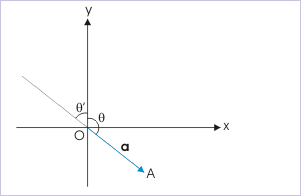Vector makes an angle with y-axis.

$\begin{array}{c}\mathrm{tan}\theta =\frac{{a}_{x}}{{a}_{y}}\end{array}$

Now, we apply the formulae to find the angle, say θ, with y-axis,

$\begin{array}{c}\mathrm{tan}\theta =-\frac{\surd 3}{1}=-\surd 3=\mathrm{tan}120°\end{array}$

$\begin{array}{c}⇒\theta =120°\end{array}$

In case, we are only interested to know the magnitude of angle between vector and y-axis, then we can neglect the negative sign,

$\begin{array}{c}\mathrm{tan}\mathrm{\theta ’}=\surd 3=\mathrm{tan}60°\end{array}$

$\begin{array}{c}⇒\mathrm{\theta ’}=60°\end{array}$

If a vector makes angles α,β and γ with x,y and z axes of a rectangular coordinate system, then prove that :

$\begin{array}{c}{\mathrm{cos}}^{2}\alpha +{\mathrm{cos}}^{2}\beta +{\mathrm{cos}}^{2}\gamma =1\end{array}$

The vector can be expressed in terms of its component as :

$\begin{array}{c}\mathbf{a}={a}_{x}\mathbf{i}+{a}_{y}\mathbf{j}+{a}_{z}\mathbf{k}\end{array}$

where ${a}_{x}=a\mathrm{cos}\alpha ;\phantom{\rule{4pt}{0ex}}{a}_{y}=a\mathrm{cos}\beta ;\phantom{\rule{4pt}{0ex}}{a}_{z}=a\mathrm{cos}\gamma$ .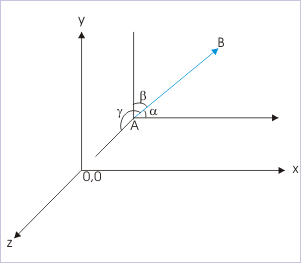Vector makes different angles with axes..

The magnitude of the vector is given by :

$\begin{array}{l}a=\surd \left({{a}_{x}}^{2}+{{a}_{y}}^{2}+{{a}_{z}}^{2}\right)\end{array}$

Putting expressions of components in the equation,

$\begin{array}{l}⇒a=\surd \left({a}^{2}{\mathrm{cos}}^{2}\alpha +{a}^{2}{\mathrm{cos}}^{2}\beta +{a}^{2}{\mathrm{cos}}^{2}\gamma \right)\end{array}$

$\begin{array}{l}⇒\surd \left({\mathrm{cos}}^{2}\alpha +{\mathrm{cos}}^{2}\beta +{\mathrm{cos}}^{2}\gamma \right)=1\end{array}$

Squaring both sides,

$\begin{array}{l}⇒{\mathrm{cos}}^{2}\alpha +{\mathrm{cos}}^{2}\beta +{\mathrm{cos}}^{2}\gamma =1\end{array}$

Find the components of weight of a block along the incline and perpendicular to the incline.

The component of the weight along the incline is :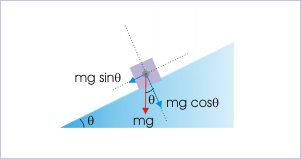Components of weight along the incline and perpendicualt to the incline.

$\begin{array}{c}{W}_{x}=\mathrm{mg}\phantom{\rule{2pt}{0ex}}\mathrm{sin}\theta =100\phantom{\rule{2pt}{0ex}}x\phantom{\rule{2pt}{0ex}}\mathrm{sin}30°=100\phantom{\rule{2pt}{0ex}}x\phantom{\rule{2pt}{0ex}}\frac{1}{2}=50\phantom{\rule{2pt}{0ex}}N\end{array}$

and the component of weight perpendicular to incline is :

$\begin{array}{c}{W}_{y}=\mathrm{mg}\phantom{\rule{2pt}{0ex}}\mathrm{cos}\theta =100\phantom{\rule{2pt}{0ex}}x\phantom{\rule{2pt}{0ex}}\mathrm{cos}30°=100\phantom{\rule{2pt}{0ex}}x\phantom{\rule{2pt}{0ex}}\frac{\surd 3}{2}=50\surd 3\phantom{\rule{2pt}{0ex}}N\end{array}$

The sum of magnitudes of two forces acting at a point is 16 N. If the resultant of the two forces is 8 N and it is normal to the smaller of the two forces, then find the forces.

We depict the situation as shown in the figure. The resultant force R is shown normal to small force ${F}_{1}$ . In order that the sum of the forces is equal to R, the component of larger force along the x-direction should be equal to smaller force :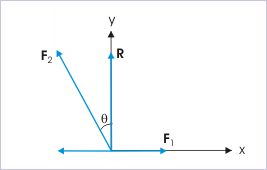The resultant force is perpendicular to smaller of the two forces.

$\begin{array}{c}{F}_{2}\mathrm{sin}\theta ={F}_{1}\end{array}$

Also, the component of the larger force along y-direction should be equal to the magnitude of resultant,

$\begin{array}{c}{F}_{2}\mathrm{cos}\theta =R=8\end{array}$

Squaring and adding two equations, we have :

$\begin{array}{c}{F}_{2}^{2}={F}_{1}^{2}+64\end{array}$

$\begin{array}{c}⇒{F}_{2}^{2}-{F}_{1}^{2}=64\end{array}$

However, according to the question,

$\begin{array}{c}⇒{F}_{1}+{F}_{2}=16\end{array}$

Substituting, we have :

$\begin{array}{c}⇒{F}_{2}-{F}_{1}=\frac{64}{16}=4\end{array}$

Solving,

$\begin{array}{c}⇒{F}_{1}=6\phantom{\rule{2pt}{0ex}}N\\ ⇒{F}_{2}=10\phantom{\rule{2pt}{0ex}}N\end{array}$

More illustrations on the subject are available in the module titled Resolution of forces

#### Questions & Answers

What are the system of units
Jonah Reply
A stone propelled from a catapult with a speed of 50ms-1 attains a height of 100m. Calculate the time of flight, calculate the angle of projection, calculate the range attained
Samson Reply
58asagravitasnal firce
Amar
water boil at 100 and why
isaac Reply
what is upper limit of speed
Riya Reply
what temperature is 0 k
Riya
0k is the lower limit of the themordynamic scale which is equalt to -273 In celcius scale
Mustapha
How MKS system is the subset of SI system?
Clash Reply
which colour has the shortest wavelength in the white light spectrum
Mustapha Reply
how do we add
Jennifer Reply
if x=a-b, a=5.8cm b=3.22 cm find percentage error in x
Abhyanshu Reply
x=5.8-3.22 x=2.58
sajjad
what is the definition of resolution of forces
Atinuke Reply
what is energy?
James Reply
Ability of doing work is called energy energy neither be create nor destryoed but change in one form to an other form
Abdul
motion
Mustapha
highlights of atomic physics
Benjamin
can anyone tell who founded equations of motion !?
Ztechy Reply
n=a+b/T² find the linear express
Donsmart Reply
أوك
عباس
Quiklyyy
Sultan Reply
Moment of inertia of a bar in terms of perpendicular axis theorem
Sultan Reply
How should i know when to add/subtract the velocities and when to use the Pythagoras theorem?
Yara Reply

### Read also:

#### Get the best Physics for k-12 course in your pocket!

Source:  OpenStax, Physics for k-12. OpenStax CNX. Sep 07, 2009 Download for free at http://cnx.org/content/col10322/1.175
Google Play and the Google Play logo are trademarks of Google Inc.

Notification Switch

Would you like to follow the 'Physics for k-12' conversation and receive update notifications?ByBy Eric CrawfordBy Edward Biton